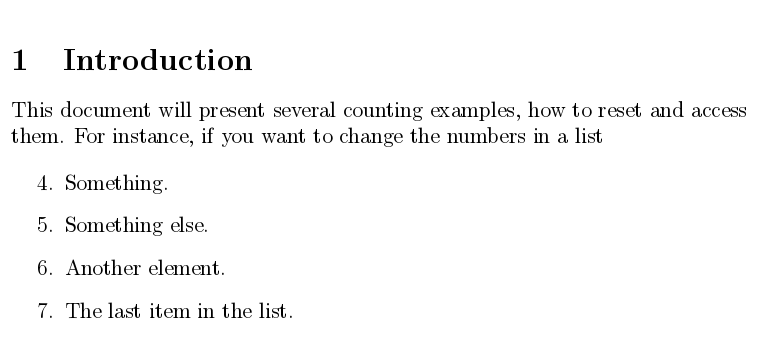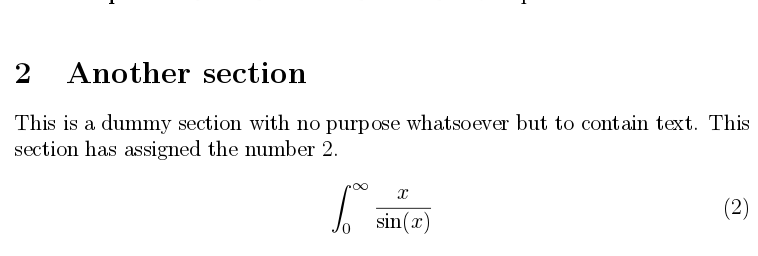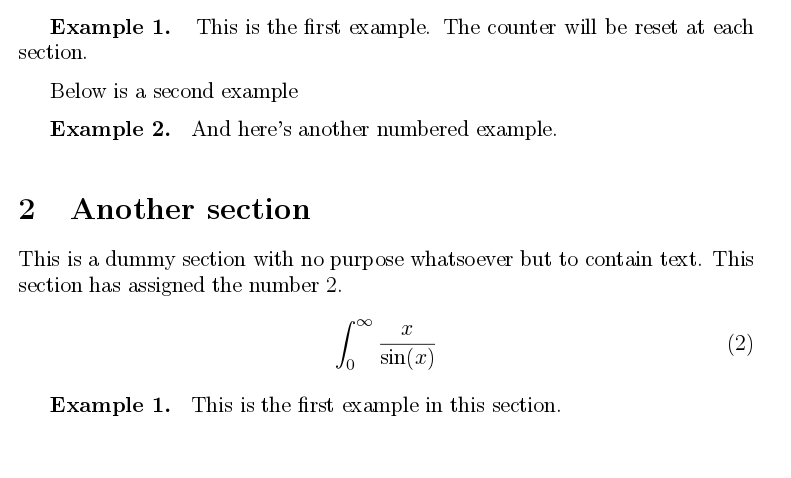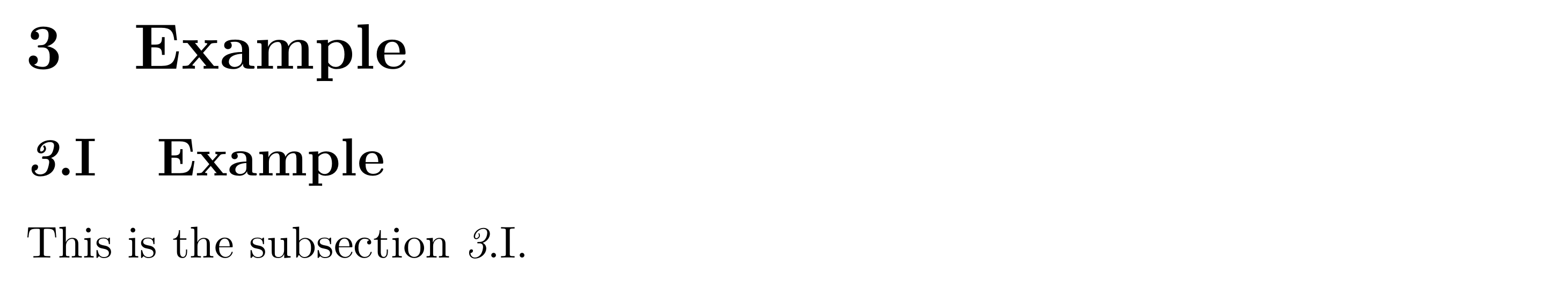Showing first {{hits.length}} results of {{hits_total}} for {{searchQueryText}}{{hits.length}} results for {{searchQueryText}}

LaTeX uses internal counters that provide numbering of pages, sections, tables, figures, etc. This article explains how to access and modify those counters and how to create new ones. Contents 1 Introduction 2 Counter manipulation 3 Creating new counters 4 Printing counters 4.1 Formatting counters 5 Reference guide 6 Further reading Introduction A counter can be easily set to any arbitrary value with \setcounter. See the example below: \section{Introduction} This document will present several counting examples, how to reset and access them. For instance, if you want to change the numbers in a list. \begin{enumerate} \setcounter{enumi}{3} \item Something. \item Something else. \item Another element. \item The last item in the list. \end{enumerate}In this example \setcounter{enumi}{3} sets the value of the item counter in the list to 3. This is the general syntax to manually set the value of any counter. See the reference guide for a complete list of counters.   Open an example in Overleaf Counter manipulation All commands changing a counter's state in this section are changing it globally. Counters in a document can be incremented, reset, accessed and referenced. Let's see an example: \section{Another section} This is a dummy section with no purpose whatsoever but to contain text. This section has assigned the number \thesection. \stepcounter{equation} \begin{equation} \label{1stequation} \int_{0}^{\infty} \frac{x}{\sin(x)} \end{equation}In this example, two counters are used: \thesection Prints 2, the value of the counter section at this point. For further methods to print a counter take a look on how to print counters. \stepcounter{equation} Increases by 1 the value of the counter equation. Other similar commands are \addtocounter and \refstepcounter, see the reference guide. Further commands to manipulate counters include: \counterwithin<*>{}{} Add to the counters that reset when they're incremented. If you don't provide the *, \the will be redefined to \the.\arabic{}. This macro is included in the LaTeX format since April 2018, if you're using an older version, you'll have to use the chngctr package. \counterwithout<*>{}{} Remove from the counters that reset when they're incremented. If you don't provide the *, \the will be redefined to \arabic{}. This macro is included in the LaTeX format since April 2018, if you're using an older version, you'll have to use the chngctr package. \addtocounter{}{} Add to the value of the counter . \setcounter{}{} Set the counter 's value to . \refstepcounter{} Like \stepcounter but you can use LaTeX's referencing system to add a \label and later \ref the counter. The printed reference will be the current expansion of \the.   Open an example in Overleaf Creating new counters The basic syntax to create a new counter is by \newcounter. Below an example that defines a numbered environment called example: \documentclass{article} \usepackage[utf8]{inputenc} \usepackage[english]{babel} \newcounter{example}[section] \newenvironment{example}[]{\refstepcounter{example}\par\medskip \textbf{Example~\theexample. #1} \rmfamily}{\medskip} \begin{document} This document will present... \begin{example} This is the first example. The counter will be reset at each section. \end{example} Below is a second example \begin{example} And here's another numbered example. \end{example} \section{Another section} This is a dummy section with no purpose whatsoever but to contain text. This section has assigned the number \thesection. \stepcounter{equation} \begin{equation} \label{1stequation} \int_{0}^{\infty} \frac{x}{\sin(x)} \end{equation} \begin{example} This is the first example in this section. \end{example} \end{document}In this LaTeX snippet the new environment example is defined, this environment has 3 counting-specific commands. \newcounter{example}[section] Creates a new counter called example that will be reset every time the section counter is increased. You can put any other counter instead of section or omit the parameter if you don't want your defined counter to be automatically reset. \refstepcounter{example} Increases the counter by 1 and makes it visible for the referencing mechanism, so that you can use \label afterwards. \theexample Prints the current value of the counter example. For further information on user-defined environments see the article about defining new environments   Open an example in Overleaf Printing counters You can print the current value of a counter in different ways: \theCounterName prints the value of the counter formatted (this is what LaTeX uses, e.g. to typeset section titles), e.g. 2.1 for the first subsection in the second section. \arabic prints the value as an Arabic number, e.g. 2. \value this one isn't meant to print the counter's state but to access it in a numeric expression (e.g. in \setcounter{section}{\value{subsection}}). \alph prints the value as an alphabetic character (minuscule), e.g. b. \Alph prints the value as an alphabetic character (capital letter), e.g. B. \roman prints the value as a Roman number (minuscules), e.g. ii. \Roman prints the value as a Roman number (capital letters), e.g. II. \fnsymbol prints the value as a symbol in a sequence, this is meant to be used for symbolic footnotes, e.g. †. Formatting counters \theCounterName is the macro responsible to print CounterName's value in a formatted manner. For new counters created by \newcounter it gets initialized as an Arabic number. You can change this by using \renewcommand. For example if you want to change the way a subsection counter is printed to include the current section in italics and the current subsection in uppercase Roman numbers, you could do the following: \renewcommand\thesubsection{\textit{\thesection}.\Roman{subsection}} \section{Example} \subsection{Example}\label{sec:example:ssec:example} This is the subsection \ref{sec:example:ssec:example}.Open an example in Overleaf Reference guide Default counters in LaTeX Usage Name For document structure part chapter section subsection subsubsection paragraph subparagraph page For floats equation figure table For footnotes footnote mpfootnote For the enumerate environment enumi enumii enumiii enumiv Counter manipulation commands Increase the value of the counter by number \addtocounter{CounterName}{number} Incrementation (increase the value of the counter by 1) \stepcounter{CounterName} Incrementation linked with referencing mechanism \refstepcounter{CounterName} It works like \stepcounter, but makes the counter visible to the referencing mechanism (\ref{label} returns counter value) Set the counter value explicitly \setcounter{CounterName}{number} Create a new counter (is automatically set to zero) \newcounter{NewCounterName} If you want the NewCounterName counter to be reset to zero every time that another OtherCounterName counter is increased, use: \newcounter{NewCounterName}[OtherCounterName] Return the value of the counter. Note, that it is not a formatted string, so it cannot be used in text but only in numeric expressions, like \setcounter{section}{\value{subsection}}. \value{CounterName} The value of the counter in a formatted string. \theCounterName for example: \thechapter, \thesection, etc. Note that this might result in more than just the counter, for example with the standard definitions of the article class \thesubsection will print Section.Subsection (e.g. 2.1).   Open an example in Overleaf Further reading For more information see: Environments Commands Lists Page numbering Sections and chapters Mathematical expressions Inserting Images Tables Typing exams in LaTeX Writing your own package Writing your own class The not so short introduction to LaTeX2ε